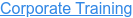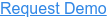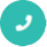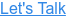#### Digital Marketing# Interview Questions for R programming

by Bhavya Sri, on May 19, 2017 4:56:01 PM## Q1. How R commands are written?

Ans: By using # at the starting of the line of code like #division commands are written.

## Q2. What is t-tests() in R?

Ans: It is used to determine that the means of two groups are equal or not by using t.test() function.

## Q3. What are the disadvantages of R Programming?

• Lack of standard GUI
• Not good for big data.
• Does not provide spreadsheet view of data.

## Q4. What is the use of With () and By () function in R?

Ans:

with() function applies an expression to a dataset.

#with(data,expression)

By() function applies a function t each level of a factors.

#by(data,factorlist,function)

## Q5. In R programming, how missing values are represented?

Ans: In R missing values are represented by NA which should be in capital letters.

## Q6. What is the use of subset() and sample() function in R?

Ans: Subset() is used to select the variables and observations and sample() function is used to  generate  a random sample of the size n from a dataset.

## Q7. Explain what is transpose.

Ans: Transpose is used for reshaping of the data which is used for analysis. Transpose is performed by t() function.

## Q8. What are the advantages of R?

Ans:

• It is used for managing and manipulating of data.
• Free and open source software.
• Graphical capabilities of R are good.
• Runs on many Operating system and different hardware and also run on 32 & 64 bit processors etc.

Now that you are aware of the benefits of R programming

## Q9. What is the function used for adding datasets in R?

Ans: For adding two datasets rbind() function is used but the column of two datasets must be same.

Syntax: rbind(x1,x2……) where x1,x2: vector, matrix, data frames.

## Q10. How you can produce co-relations and covariances?

Ans: Cor-relations is produced by cor() and covariances is produced by cov() function.

## Q11. What is difference between matrix and dataframes?

Ans: Dataframe can contain different type of data but matrix can contain only similar type of data.

## Q12. What is difference between lapply and sapply?

Ans: lapply is used to show the output in the form of list whereas sapply is used to show the output in the form of vector or data frame

## Q13. What is the difference between seq(4) and seq_along(4)?

Ans: Seq(4) means vector from 1 to 4 (c(1,2,3,4)) whereas seq_along(4) means a vector of the  length(4) or 1(c(1)).

## Q14. Explain how you can start the R commander GUI.

Ans: rcmdr command is used to start the R commander GUI.

## Q15. What is the memory limit of R?

Ans: In 32 bit system memory limit is 3Gb but most versions limited to 2Gb and in 64 bit system memory limit is 8Tb.

## Q16. How many data structures R has?

Ans: There are 5 data structure in R i.e. vector, matrix, array which are of homogenous type and other two are list and data frame which are heterogeneous.

## Q17. Explain how data is aggregated in R.

Ans: There are two methods that is collapsing data by using one or more BY variable and other is aggregate() function in which BY variable should be in list.

## Q18.  Explain how you can start the R commander GUI?

Ans: Typing the command, (“Rcmdr”) into the R console starts the R commander GUI.

## Q19. What are R packages?

Ans: Packages are the collections of data, R functions and compiled code in a well-defined format and these packages are stored in library.

## Q20. What is the workspace in R?

Ans: Workspace is the current R working environment which includes any user defined objects like vector, lists etc.

## Q21. What is the function which is used for merging of data frames horizontally in R?

Ans: Merge()function is used to merge two data frames
Eg. Sum<-merge(data frame1,data frame 2,by=’ID’.

## Q22. what is the function which is used for merging of data frames vertically in R?

Ans: rbind() function is used to merge two data frames vertically.
Eg.

Sum<- rbind(data frame1,data frame 2)

## Q23. What is the power analysis?

Ans: It is used for experimental design .It is used to determine the effect of given sample size.

## Q24. Which package is used for power analysis in R?

Ans: Pwr package is used for power analysis in R.

## Q25. Which method is used for exporting the data in R?

Ans: There are many ways to export the data into another formats like SPSS, SAS , Stata , Excel Spreadsheet.

## Q26. Which packages are used for exporting of data?

Ans: For excel xlsReadWrite package is used and for sas,spss ,stata foreign package is implemented.

## Q27. How impossible values are represented in R?

Ans: In R NaN is used to represent impossible values.

## Q28. Which command is used for storing R object into a file?

Ans: Save command is used for storing R objects into a file.
Syntax: >save(z,file=”z.Rdata”)

## Q29. Which command is used for restoring R object from a file?

Ans: load command is used for storing R objects from a file.

## Q30. What is the use of coin package in R?

Ans: Coin package is used to achieve the re randomization or permutation based statistical tests.

## Q31. Which function is used for sorting in R?

Ans: order() function is used to perform the sorting.

Ans: IOS-6.1.3

## Q33. What happens when the application object does not handle an event?

Ans: The event will be dispatched to your delegate for processing.

## Q34. Explain app specific objects which store the app contents.

Ans: The app specific objects are Data model objects that store app’s contents.

## Q35. Explain the purpose of using UIWindow object?

Ans: UIWindow object coordinates the one or more views presenting on the screen.

## Q36. Tell me the super class of all view controller objects.

Ans: UIView Controller class.

## Q37. How to create axes in the graph?

Ans: Using axes() function custom axes are created.

## Q38. What is the use of abline() function?

Ans: abline() function is add the reference line to a graph.
Syntax:-

abline(h=yvalues, v=xvalues)

## Q39. Why vcd package is used?

Ans: vcd package provides different methods for visualizing multivariate categorical data.

## Q40. What is GGobi?

Ans: GGobi is an open source program for visualization for exploring high dimensional typed data.

## Q41. What is iPlots?

Ans: It is a package which provide bar plots, mosaic plots, box plots, parallel plots, scatter plots and histograms.

## Q42. What is the use of lattice package?

Ans: lattice package is to improve on base R graphics by giving better defaults and it have the ability to easily display multivariate relationships.

## Q43. What is fitdistr() function?

Ans: It is used to provide the maximum likelihood fitting of univariate distributions. It is defined under the MASS package.

## Q44. Which data structures are used to perform statistical analysis and create graphs.

Ans: Data structures are vectors, arrays, data frames and matrices.

## Q45. What is the use of sink() function?

Ans: It defines the direction of output.

## Q46. Why library() function is used?

Ans: This function is used to show the packages which are installed.

## Q47. Why search() function is used?

Ans: By this function we see that which packages are currently loaded.

## Q48. On which type of data binary operators are worked?

Ans: Binary operators are worked on matrices, vectors and scalars.

## Q49. What is the use of doBY package?

Ans: It is used to define the desired table using function and model formula.

## Q50. Explain how you can start the R commander GUI?

Ans: Typing the command, (“Rcmdr”) into the R console starts the R commander GUI.